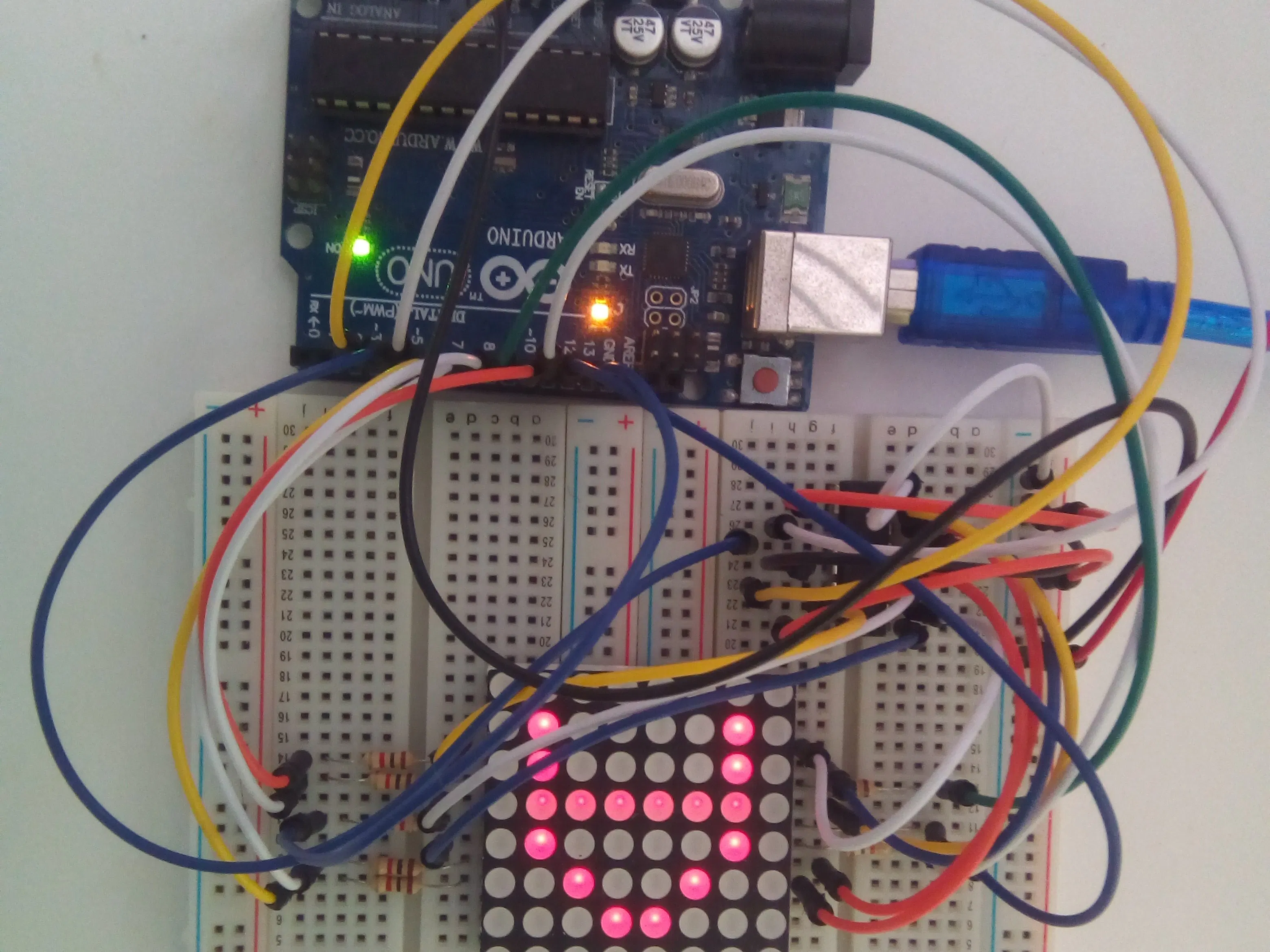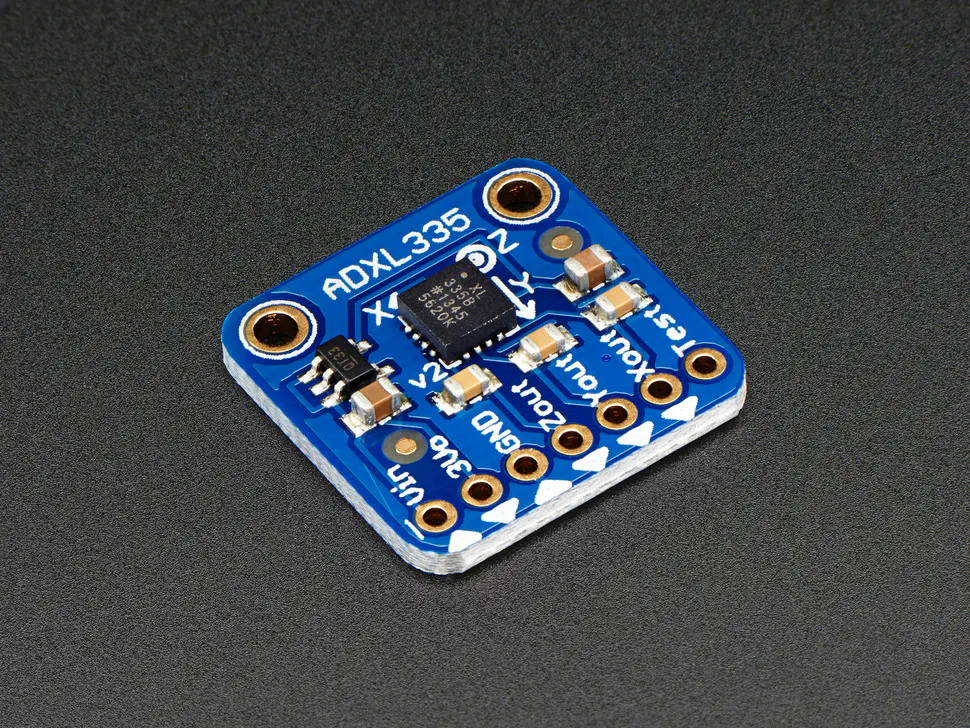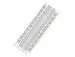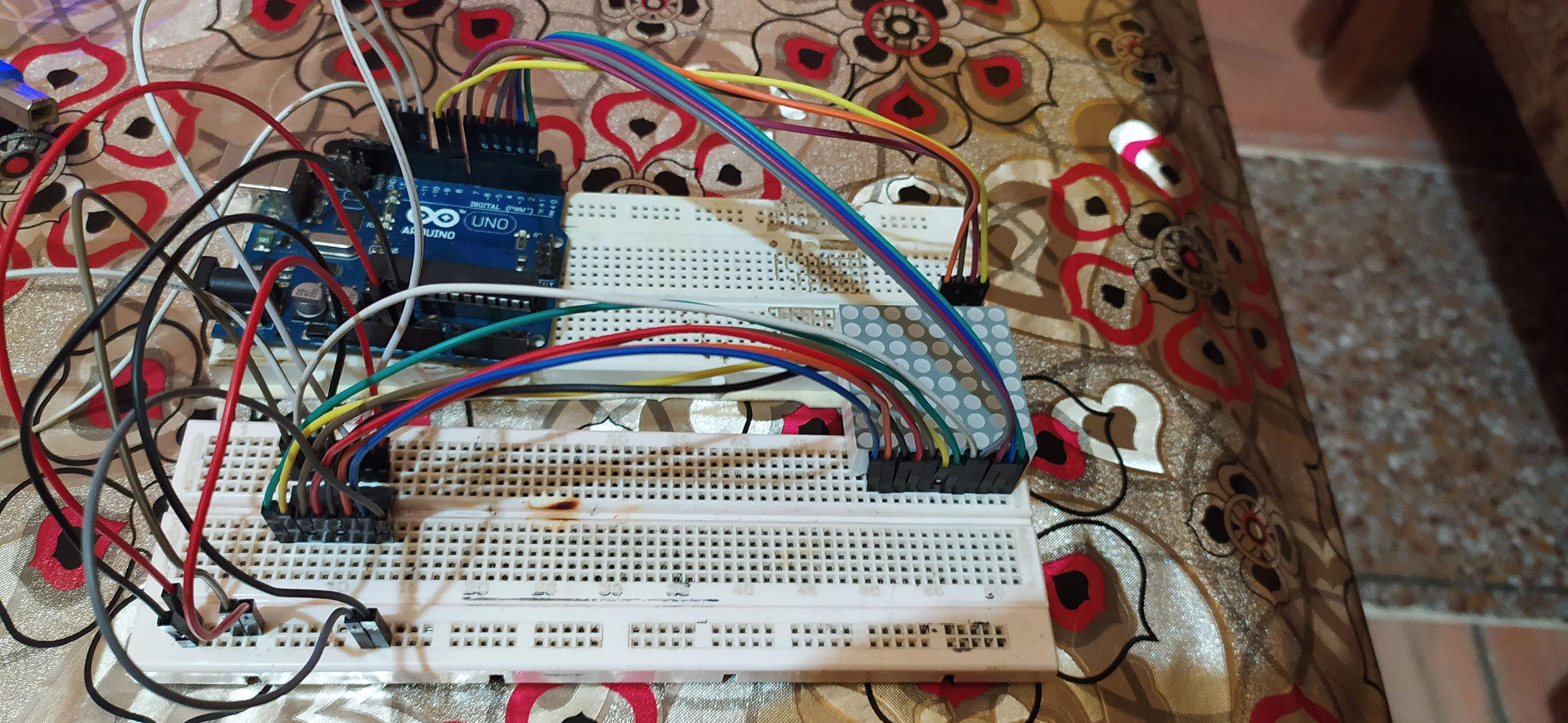Published

Tilt to Tell

Exciting project to display the direction of tilt using 8x8 led matrix.Things used in this project

Hardware componentsArduino UNO
×1
 8x8 led matrix
×1
 74HC595 shift register
×1×1Jumper wires (generic)
×25×1

Software apps and online servicesArduino IDE

Schematics

CircuitCode

Arduino code

Arduino
int i,j,t=100,dpin=13,lpin=12,cpin=11,a,b,c;
int r={2,3,4,5,6,7,8,9};
byte up={B11100111,
B11000011,
B10000001,
B00000000,
B11100111,
B11100111,
B11100111,
B11100111
};
byte down={B11100111,
B11100111,
B11100111,
B11100111,
B00000000,
B10000001,
B11000011,
B11100111
};
byte left={B11101111,
B11001111,
B10001111,
B00000000,
B00000000,
B10001111,
B11001111,
B11101111
};
byte right={B11110111,
B11110011,
B11110001,
B00000000,
B00000000,
B11110001,
B11110011,
B11110111
};
byte ur={B11110000,
B11111100,
B11111010,
B11110110,
B11101111,
B11011111,
B10111111,
B01111111
};
byte ul={B00001111,
B00111111,
B01011111,
B01101111,
B11110111,
B11111011,
B11111101,
B11111110
};
byte dr={B01111111,
B10111111,
B11011111,
B11101111,
B11110110,
B11111010,
B11111100,
B11110000
};
byte dl={B11111110,
B11111101,
B11111011,
B11110111,
B01101111,
B01011111,
B00111111,
B00001111
};
byte o={B11111111,
B11111111,
B11111111,
B11100111,
B11100111,
B11111111,
B11111111,
B11111111
};
byte no={B01111110,
B10111101,
B11011011,
B11100111,
B11100111,
B11011011,
B10111101,
B01111110
};
void setup()
{
pinMode(dpin,OUTPUT);
pinMode(lpin,OUTPUT);
pinMode(cpin,OUTPUT);
pinMode(a,INPUT);
pinMode(b,INPUT);
pinMode(c,INPUT);
for( i=0;i<8;i++)
{
pinMode(r[i],OUTPUT);
digitalWrite(r[i],LOW);
}
}
void disp(byte ch)
{
for(i=0;i<8;i++)
{
digitalWrite(r[(7-i)],HIGH);
digitalWrite(lpin,LOW);
shiftOut(dpin,cpin,MSBFIRST,ch[i]);
digitalWrite(lpin,HIGH);

digitalWrite(lpin,LOW);
shiftOut(dpin,cpin,MSBFIRST,B11111111);
digitalWrite(lpin,HIGH);
digitalWrite(r[(7-i)],LOW);
}
}
void loop()
{
int x,y,z;
if(z<90)
{
if(x<-10 && (y>-10 && y<10))
for(j=0;j<t;j++)
disp(up);
else if(x>10 && (y>-10 && y<10))
for(j=0;j<t;j++)
disp(down);
else if(y<-10 && (x>-10 && x<10))
for(j=0;j<t;j++)
disp(left);
else if(y>10 && (x>-10 && x<10))
for(j=0;j<t;j++)
disp(right);
else if(x<-10 && y<-10)
for(j=0;j<t;j++)
disp(ul);
else if(x>10 && y<-10)
for(j=0;j<t;j++)
disp(dl);
else if(x<-10 && y>10)
for(j=0;j<t;j++)
disp(ur);
else if(x>10 && y>10)
for(j=0;j<t;j++)
disp(dr);
else if((x<10 && x>-10)&& (y>-10 && y<10))
for(j=0;j<t;j++)
disp(o);
}
else
disp(no);
}